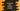# JavaScript Map forEach method example## JavaScript Map forEach method example:

Map is a built-in object in JavaScript. Map object is used to hold key-value pairs. We can insert key-value pairs and using the key, we can access the value associated with it. Map also remembers the insertion order of the pairs.

## forEach method:

forEach method is used to execute a given function over each pair of the map. It runs the function in the same order the pairs are inserted.

This method is defined as like below:

``forEach(callBack, arg)``

Here,

• callBack is the function to execute on each key-value pairs. It can be an arrow function or any normal javascript function. This function takes the following optional arguments:

• value: This is an optional value. It is the value in each iteration.
• key: This is an optional value. It is the key in each iteration.
• map: This is also optional value. It is the map currently iterating.

arg is an optional value, it is used as this while executing callbacks.

### Return value of forEach:

forEach method doesn’t return anything or it returns undefined.

## Example of forEach:

Let’s take an example of forEach to print all key-value pairs of a map:

``````let givenMap = new Map();

givenMap.set('one', 1);
givenMap.set('two', 2);
givenMap.set('three', 3);
givenMap.set('four', 4);
givenMap.set('five', 5);

function printData(value, key, map){
console.log(`Key: \${key}, Value: \${value}`);
}
givenMap.forEach(printData);``````

In this example,

• givenMap is a Map object and we inserted five different key-value pairs.
• printData function is used to print the key and value.
• printData is passed to forEach.

If you run this program, it will print the below output:

``````Key: one, Value: 1
Key: two, Value: 2
Key: three, Value: 3
Key: four, Value: 4
Key: five, Value: 5``````

## Example of forEach with inline function:

We can use an inline function. For example:

``````let givenMap = new Map();

givenMap.set('one', 1);
givenMap.set('two', 2);
givenMap.set('three', 3);
givenMap.set('four', 4);
givenMap.set('five', 5);

givenMap.forEach(function printData(value, key, map){
console.log(`Key: \${key}, Value: \${value}`);
});``````

It will give similar output.

## Example of forEach with an arrow function:

We can also use one arrow function.

``````let givenMap = new Map();

givenMap.set('one', 1);
givenMap.set('two', 2);
givenMap.set('three', 3);
givenMap.set('four', 4);
givenMap.set('five', 5);

givenMap.forEach((value, key, map)=> {
console.log(`Key: \${key}, Value: \${value}`);
});``````

You will get the same result.